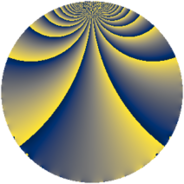# Properties

 Label 512.2.aLevel $512$ Weight $2$ Character orbit 512.a Rep. character $\chi_{512}(1,\cdot)$ Character field $\Q$ Dimension $16$ Newform subspaces $7$ Sturm bound $128$ Trace bound $7$

# Related objects

## Defining parameters

 Level: $$N$$ $$=$$ $$512 = 2^{9}$$ Weight: $$k$$ $$=$$ $$2$$ Character orbit: $$[\chi]$$ $$=$$ 512.a (trivial) Character field: $$\Q$$ Newform subspaces: $$7$$ Sturm bound: $$128$$ Trace bound: $$7$$ Distinguishing $$T_p$$: $$3$$, $$5$$, $$7$$

## Dimensions

The following table gives the dimensions of various subspaces of $$M_{2}(\Gamma_0(512))$$.

Total New Old
Modular forms 80 16 64
Cusp forms 49 16 33
Eisenstein series 31 0 31

The following table gives the dimensions of the cuspidal new subspaces with specified eigenvalues for the Atkin-Lehner operators and the Fricke involution.

$$2$$Dim.
$$+$$$$6$$
$$-$$$$10$$

## Trace form

 $$16q + 16q^{9} + O(q^{10})$$ $$16q + 16q^{9} + 16q^{25} + 16q^{49} - 32q^{65} - 32q^{73} + 16q^{81} - 32q^{89} + O(q^{100})$$

## Decomposition of $$S_{2}^{\mathrm{new}}(\Gamma_0(512))$$ into newform subspaces

Label Dim. $$A$$ Field CM Traces A-L signs $q$-expansion
$$a_2$$ $$a_3$$ $$a_5$$ $$a_7$$ 2
512.2.a.a $$2$$ $$4.088$$ $$\Q(\sqrt{2})$$ $$\Q(\sqrt{-2})$$ $$0$$ $$-4$$ $$0$$ $$0$$ $$+$$ $$q+(-2+\beta )q^{3}+(3-4\beta )q^{9}+(-2-3\beta )q^{11}+\cdots$$
512.2.a.b $$2$$ $$4.088$$ $$\Q(\sqrt{2})$$ None $$0$$ $$0$$ $$-4$$ $$0$$ $$+$$ $$q+\beta q^{3}-2q^{5}-2\beta q^{7}-q^{9}-3\beta q^{11}+\cdots$$
512.2.a.c $$2$$ $$4.088$$ $$\Q(\sqrt{2})$$ None $$0$$ $$0$$ $$0$$ $$-8$$ $$+$$ $$q+\beta q^{3}-2\beta q^{5}-4q^{7}-q^{9}+\beta q^{11}+\cdots$$
512.2.a.d $$2$$ $$4.088$$ $$\Q(\sqrt{2})$$ None $$0$$ $$0$$ $$0$$ $$8$$ $$-$$ $$q+\beta q^{3}+2\beta q^{5}+4q^{7}-q^{9}+\beta q^{11}+\cdots$$
512.2.a.e $$2$$ $$4.088$$ $$\Q(\sqrt{2})$$ None $$0$$ $$0$$ $$4$$ $$0$$ $$-$$ $$q+\beta q^{3}+2q^{5}+2\beta q^{7}-q^{9}-3\beta q^{11}+\cdots$$
512.2.a.f $$2$$ $$4.088$$ $$\Q(\sqrt{2})$$ $$\Q(\sqrt{-2})$$ $$0$$ $$4$$ $$0$$ $$0$$ $$-$$ $$q+(2+\beta )q^{3}+(3+4\beta )q^{9}+(2-3\beta )q^{11}+\cdots$$
512.2.a.g $$4$$ $$4.088$$ $$\Q(\zeta_{24})^+$$ None $$0$$ $$0$$ $$0$$ $$0$$ $$-$$ $$q+\beta _{1}q^{3}-\beta _{2}q^{5}-\beta _{3}q^{7}+3q^{9}+\beta _{1}q^{11}+\cdots$$

## Decomposition of $$S_{2}^{\mathrm{old}}(\Gamma_0(512))$$ into lower level spaces

$$S_{2}^{\mathrm{old}}(\Gamma_0(512)) \cong$$ $$S_{2}^{\mathrm{new}}(\Gamma_0(32))$$$$^{\oplus 5}$$$$\oplus$$$$S_{2}^{\mathrm{new}}(\Gamma_0(64))$$$$^{\oplus 4}$$$$\oplus$$$$S_{2}^{\mathrm{new}}(\Gamma_0(128))$$$$^{\oplus 3}$$$$\oplus$$$$S_{2}^{\mathrm{new}}(\Gamma_0(256))$$$$^{\oplus 2}$$